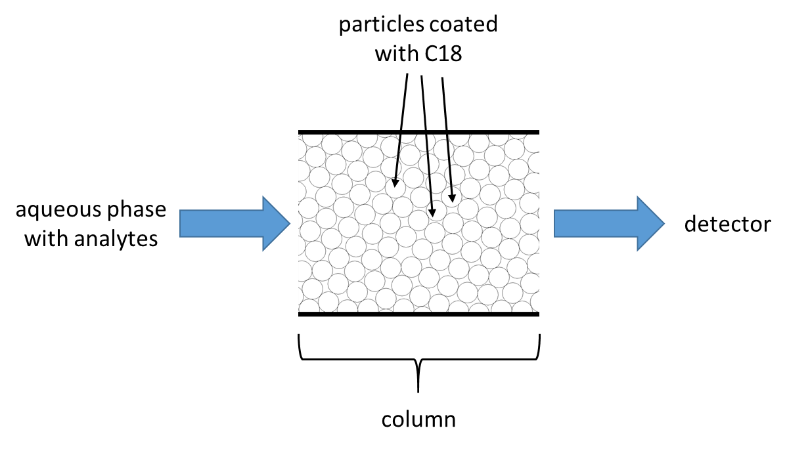# Partition Coefficient and Chromatographic Retention Order

In-Class Activity: Introduction to Separations

Learning Objectives:

At the completion of this activity, you should be able to

• Identify the chemical/physical property that controls separation of compounds in a given scenario
• Relate the concept of partition coefficients to the separation of compounds on a column
• Predict elution order in separations of compounds in liquid chromatography

For the first three questions in this activity, you’ll have to think way back to some things you learned in organic chemistry.  If you have not yet had organic chemistry, ask the other members of your group to explain how an extraction works.

1. You have a sample that consists of water with a complex mixture of trace levels of organic compounds. The compounds can be grouped into broad categories of organic acids, organic bases and neutral organics.  Which functional groups are found in organic acids?  Which functional groups are found in organic bases?

2. What do these functional groups look like at very low or very high pH values?

3. Devise a way to separate the materials in the sample described above by performing an extraction. The desire is to have three solutions at the end, each in methylene chloride, one of which contains only the organic acids, the second contains only the organic bases, and the third contains only the neutrals.

Remember:

• Ions are more soluble in water than in organic solvents.
• Neutrals are more soluble in organic solvents than in water.

Extractions are rarely complete – 100% of the compound is not extracted from one phase to the other.  The relative affinity of the compound for one phase over the other will determine how much of the compound is found in each phase.

1. Imagine a two-phase system consisting of octanol (C8H17OH) and water. Which chemical property/ies do you think would control the affinity of a compound for each of these phases?

If we assume that an equilibrium is established as a compound partitions between the two phases, we can write an equilibrium expression that describes this partitioning. The log of the value of these equilibrium constants, or octanol-water partition coefficients, for several drug components are shown below:1. Which of the equations given below is the correct expression for Kow? How do you know?

$K_{ow} = \dfrac{[X]_{octanol}}{[X]_{water}} \hspace{50px} K_{ow} = \dfrac{[X]_{water}}{[X]_{octanol}}\nonumber$

2. Assuming that you added equal molar concentrations of each of the compounds to the octanol-water system, which compound would have the highest concentration in the octanol phase? Which would have the highest concentration in the aqueous phase?

3. What – if anything – can you say about the relative value of Kow for 1,3,5-benzenetriol?1,3,5-benzenetriol

Now let’s suppose that instead of a two-phase liquid system of octanol and water, we have liquid water and a solid support material that has long hydrocarbon chains (18 carbons; C18) attached to it as shown below.1. Which chemical property/properties do you think will control the affinity of a compound for this material as compared to the aqueous phase?

1. Returning to the compounds given in the table in the previous section, predict the relative order of the partition coefficients that describe the partitioning of each compound between the material shown above and the aqueous phase (K =[X]C18/[X]aq). Rank the compounds in order from largest to smallest partition coefficient.

Finally, imagine that little particles coated with the C18 material are packed into a column, and the aqueous phase flows through it as shown below.1. A mixture of the compounds is injected into the flowing (mobile) aqueous phase. As the compounds move down the column past the (stationary) solid particles, which compound will spend the most time stuck to the stationary phase?  Which compound will spend the least amount of time stuck to the stationary phase?

2. Which compound will emerge (elute) from the end of the column first? Which one is last?